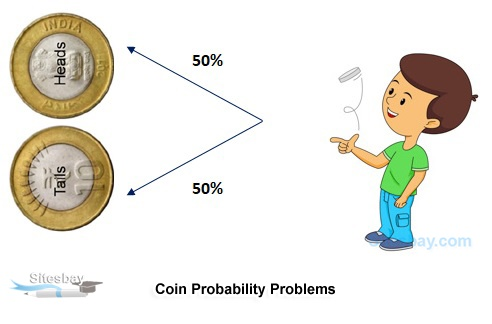# Coin Probability Problems

## Coin Probability Problems

Coin is a currency token which has two faces, one is head and other is tail. So, when throw a coin in air and when it lands it might have either a head or tail. Coin questions can be three types as shown below:### One Coint Toss

When a coin is tossed is only once then there can be two outcomes either a head or a tail. In such cases, total events = 2

Question: What is the probability of getting a head in a toss?

## Example

``` Concerned event = 1(One head)
Total Event = 2
P(E) = 1/2
```

### Two Coins Toss or One Coin Twice Toss

When two coins are tossed together or one coin is tossed in twice then following outcomes can be obtained.

• Here, 'H' = Head; 'T' = Tail.
• (H,T) shows that on coin 1 it's Head while on coin 2 it's Tail.
• Here, we can see that Total Event = 4

Question: What is the Probability of getting at most one head on tossing a coin?

## Example

```At most one head means there can be 0 head or there can be 1 head.

So, Concerned Event = 3 {(H,T) (T,H) (T,T)}
Total Events = 4
P(E) = 3/4
```

### Three Coins or One Coin Thrice

When three coins are tossed together or one coin is tossed in thrice then following outcomes can be obtained

• Here, 'H' = Head; 'T' = Tail.
• (HHH), (HHT), (HTH), (THH), (HTT), (THT), (TTH), (TTT)
• Here, (HTH) shows that coin 1 has a head, coin 2 has a Tail while coin 3 has a head.
• In such cases, Total Events = 8

Three unbiased coins are tossed. What is the probability of getting at least 2 heads?

## Formula

```Here, at least heads means there can be 2 heads and 3 heads.
So, Concerned Events = 4 {(HHH), (HHT), (HTH), (THH)}
Total Events = 8
P(E) =4/8 =1/2
```